MORE IN Mechanical Vibrations
VTU Mechanical Engineering (Semester 7)
Mechanical Vibrations
December 2014
Total marks: --
Total time: --
INSTRUCTIONS
(1) Assume appropriate data and state your reasons
(2) Marks are given to the right of every question
(3) Draw neat diagrams wherever necessary

1 (a) Explain phenomena beats
5 M
1 (b) Derive an equation for work done by harmonic force
5 M
1 (c) Represent the periodic motion given in the Fig.Q.1(c) by harmonic series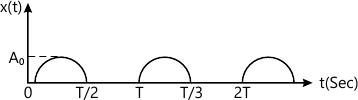10 M

2 (a) Determine the natural frequency of the system shown in the Fig.Q.2(a) by Newton's method and energy method.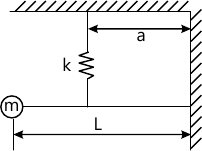10 M
2 (b) Determine the natural frequency of the system shown in the Fig.Q.2(b) by Newton's method and energy method.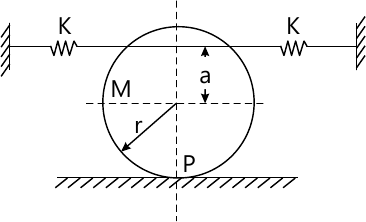10 M

3 (a) Set up the differential equation for a spring mass damper system and obtain complete solution for the critically damped condition
10 M
3 (b) Determine: i) Critical damping coefficient; ii) Damping factor; iii) Natural frequency of damped vibrations; iv) Logarithmic decrement; v) Ratio of two consecutive amplitudes of vibrating system which consists of mass of 25kg, a spring of stiffness 1 5kN/m and a damper. The damping provided is only 15% of the critical value
10 M

4 (a) Define transmissibility and derive an expression for the transmissibility ratio and the phase angle for transmitted force
10 M
4 (b) A machine of mass one ton is acted upon by an external force 2450N at a frequency of 1500RPM. To reduce the effects of vibration, isolator of rubber having a static deflection of 2mm under the machine load and an estimated damping factor of 0.2 are used. Determine: i) Force transmitted to the foundation; ii) Amplitude of vibration of the machine; iii) phase lag of the transmitted force with respect to the extremal force
10 M

5 (a) Explain Frahm's reed tachometer.
5 M
5 (b) Explain vibrometer.
5 M
5 (c) The rotor of a turbo-super charger weighing 88.3N is keyed to the centre of a 25mm diameter shaft 40cm between the bearings. Determine: i) the critical.speed of shaft ; ii) The amplitude of vibration of the rotor at a speed of 3200 RPM of the eccentricity is 0.015mm and iii) Vibratory force transmitted to the bearings at this speed. Assume the shaft to be simply supported and the shaft material has a density of 8gm/cm3. Take E :2.06× 105 Mpa
10 M

6 (a) Explain the working principle of dynamic absorber.
8 M
6 (b) Determine the natural frequencies of the spring mass pulley system as shown in the Fig.Q.6(b). The cord is inextensible and there is no slippage between the cord and the pulley. Take K1=40N/m, lK2= 60N/m; m=2kg and M- 10kg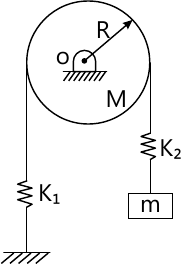12 M

7 Determine the natural frequency of the system shown in the Fig.Q.7 by HolZer's method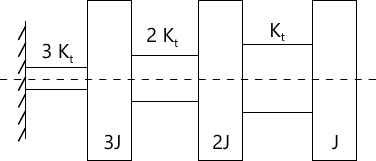20 M

8 (a) Explain the experimental modal analysis and the necessary basic equipments.
10 M
8 (b) Explain machine condition monitoring techniques.
10 M

More question papers from Mechanical Vibrations